## Random Walk--2-D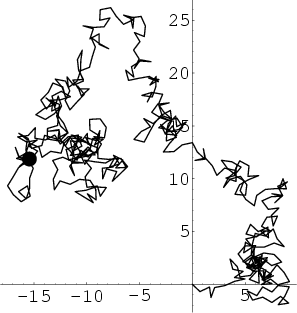In a Plane, consider a sum of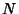2-D Vectors with random orientations. Use Phasor notation, and let the phase of each Vector be Random. Assumeunit steps are taken in an arbitrary direction (i.e., with the angleuniformly distributed inand not on a Lattice), as illustrated above. The position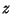in the Complex Plane aftersteps is then given by(1)

which has Absolute Square(2)

Therefore,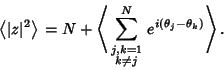(3)

Each step is likely to be in any direction, so both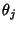andare Random Variables with identical Means of zero, and their difference is also a random variable. Averaging over this distribution, which has equally likely Positive and Negative values yields an expectation value of 0, so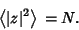(4)

The root-mean-square distance afterunit steps is therefore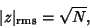(5)

so with a step size of, this becomes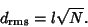(6)

In order to travel a distance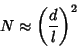(7)

steps are therefore required.Amazingly, it has been proven that on a 2-D Lattice, a random walk has unity probability of reaching any point (including the starting point) as the number of steps approaches Infinity.